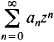Also found in: Wikipedia.

[′rād·ē·əs əv kən′vər·jəns]
(mathematics)
The positive real number corresponding to a power series expansion about some number a with the property that if x-a has absolute value less than this number the power series converges at x, and if x-a has absolute value greater than this number the power series diverges at x.

The radius of convergence of a power series is the radius of the circle of convergence. In other words, it is the number r such that the power seriesconverges when ǀzǀ > r and diverges when ǀzǀ > r.

References in periodicals archive ?
Studies have shown the dependence of the shape of the radius of convergence from the nature of the convergence.
The important characteristic of a DDM is the radius of convergence of the approximating polynomials.
Conjecture 8 For a [greater than or equal to] -1, the radius of convergence of Q(a, *) is
By Pringsheim's theorem, the radius of convergence [rho] > 0 is always a dominant singularity (7).
Armed with the plots, she and her students examined the radius of convergence, the effect of the number of terms used, the order of magnitude of the error term, and characteristics of the specific terms that appeared.
A first dichotomy emerges: when C(z) is finite at its radius of convergence [rho], the properties of A are qualitatively the same as in the 2-connected case (for which C([rho]) is known to converge), except that the limit of [p.
Compton showed that if the radius of convergence [[rho].
The lattice-dependent value [mu] is known as the growth constant, and is the reciprocal of the radius of convergence of the generating function C(x).
0] is not precised, it is by default the radius of convergence of f(z)).
Simple computations show that t(z) = 1/4 (1 - [square root of 1 - 8z]), the radius of convergence is [rho] = 1/8, t(z) has unique square root type singularity in [rho] and t([rho]) = 1/4
eta]] is the number of objects of size n: Pr([gamma]) = x|[gama]|/C(x), with x in the range ]0, [rho][, where p is the radius of convergence of C(z).
i) The radius of convergences of the series is [greater than or equal to] 1

Site: Follow: Share:
Open / Close